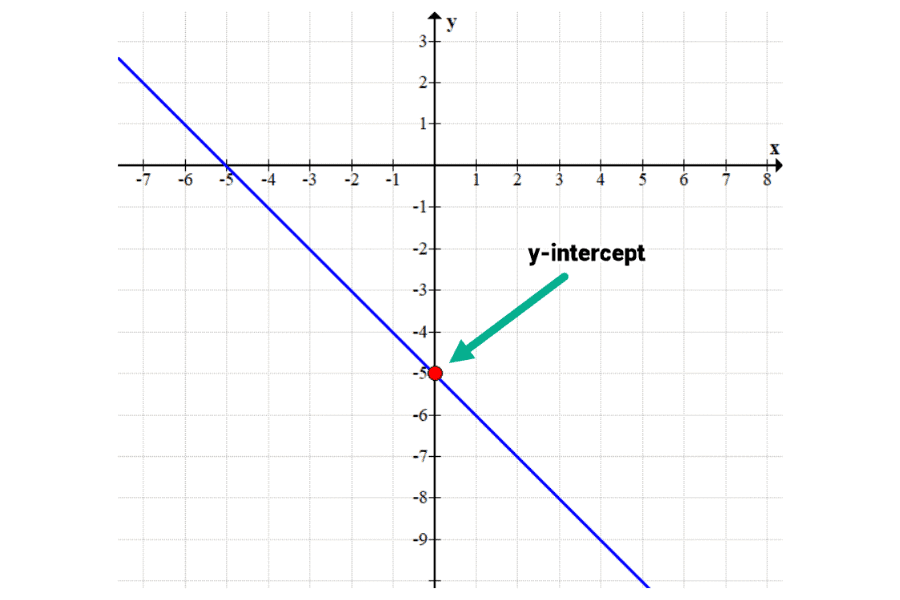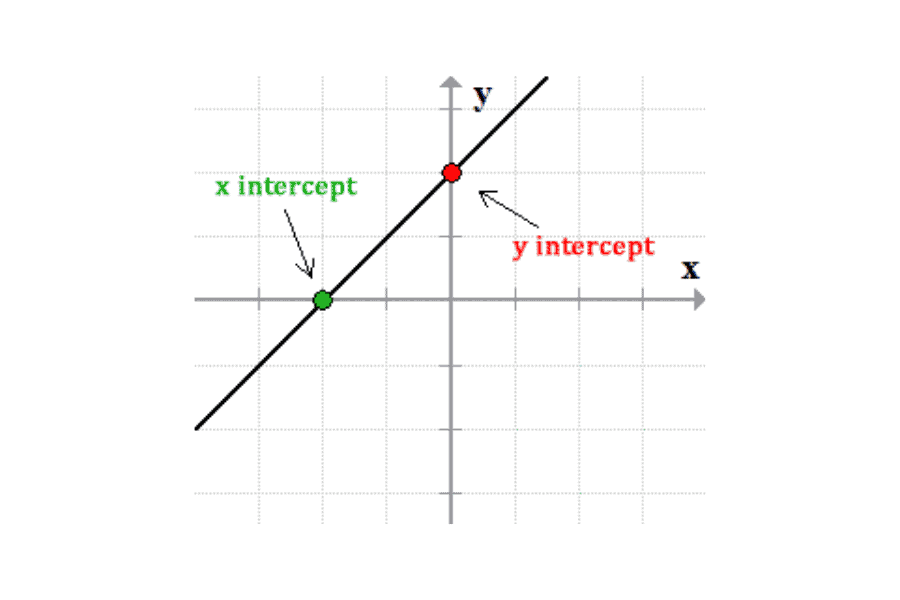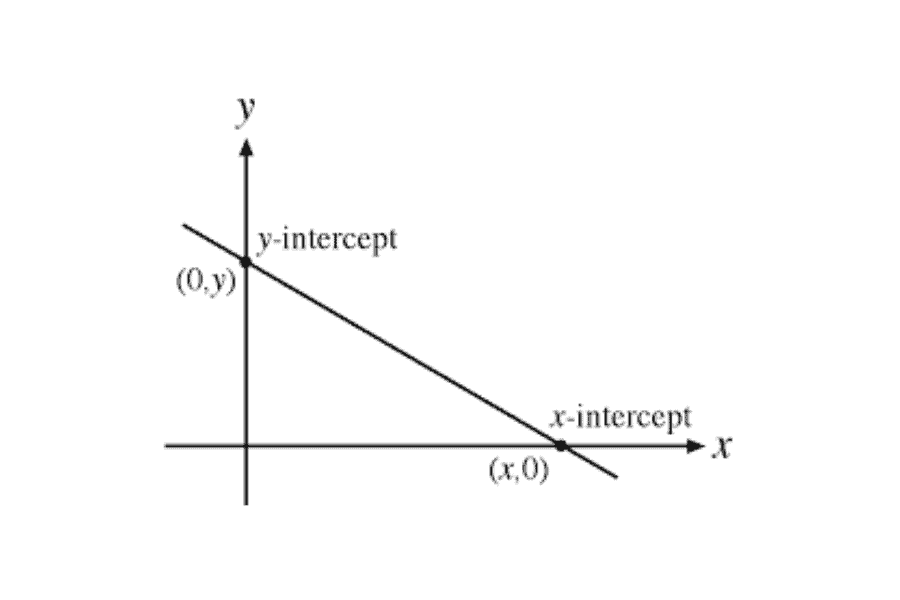# What is the Y Intercept?

In coordinate geometry, you’ll learn about lines and where the points where the cross on a graph. The point where the line crosses either the x or y axis on a graph is called an intercept.

Being able to find the intercept of a line is important to know when you’re dealing with math and science.

What is the Y Intercept?

The y-intercept is the y- value of the point where the line intersects with the y-axis. The y-intercept is also referred to as the y-value.